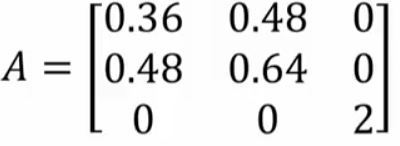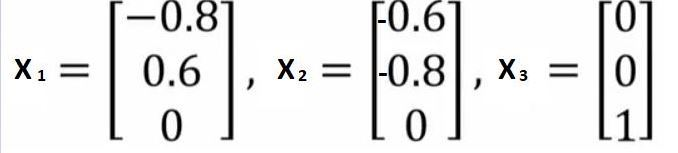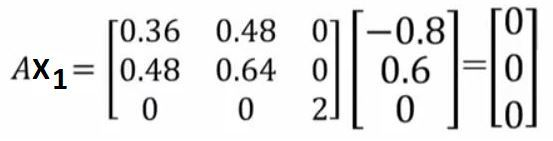Related Articles
Connection Between Eigenvectors and Nullspace
• Last Updated : 04 May, 2020

Prerequisites:

Some important points about eigenvalues and eigenvectors:

• Eigenvalues can be complex numbers even for real matrices.
• When eigenvalues become complex, eigenvectors also become complex.
• If the matrix is symmetric (e.g A = AT), then the eigenvalues are always real.
• As a result, eigenvectors of symmetric matrices are also real.
• There will always be n linearly independent eigenvectors for symmetric matrices.

Now, let’s discuss the connection between eigenvectors and nullspace.

AX = λX

Now let me ask you a question. What happens when lambda is 0? That is one of the eigenvalues becomes 0.
So, when one of the eigenvalues becomes 0, then we have this equation which is given by

AX = 0 —(equation 1)

AB = 0 —(equation 2)

So you notice that equation 1 and equation 2 form are the same.

So, that basically means that X which is an eigenvector corresponding to eigenvalue, lambda equals to 0, is a null space vector, because it is just of the form that we have noticed here. So, we could say, the eigenvectors corresponding to zero eigenvalues are in the null space of the original matrix A. Conversely, if the eigenvalue corresponding to an eigenvector is not 0, then that eigenvector can not be in the null space of A. So, these are important results that we need to know.
So, this is how eigenvectors are connected to nullspace.

Example:
Consider the following A matrixNotice that this is a symmetric matrix hence the eigenvalues are always real as I told before in the important points section.
The eigenvalues for this matrix are

λ = (0, 1, 2)

The eigenvectors corresponding to these eigenvalues areCode: Python code to calculate eigenvalue and eigenvector

 `# Python program to illustrate ` `# connection between eigenvectors and nullspace ` ` `  `# Importing required libraries ` `import` `numpy as np ` `from` `numpy ``import` `linalg ` ` `  `# Taking A matrix ` `A ``=` `np.array([ ` `    ``[``0.36``, ``0.48``, ``0``], ` `    ``[``0.48``, ``0.64``, ``0``], ` `    ``[``0``, ``0``, ``2``] ` `]) ` ` `  `# Calculating eigenvalues and eigenvectors ` `eigValues, eigVectors ``=` `linalg.eig(A) ` ` `  `# Printing those values ` `print``(``"Eigenvalue are :"``, eigValues) ` `print``(``"Eigenvectors are :"``, eigVectors) ` ` `  `# Taking eigenvector 1 ` `eigVector1 ``=` `np.array([ ` `    ``[``-``0.8``], ` `    ``[``0.6``], ` `    ``[``0``] ` `]) ` ` `  `# Matrix multiplication between A and eigenvector1 ` `result ``=` `np.dot(A, eigVector1) ` `# Print the result ` `print``(result) ` ` `  `# This code is contributed by Amiya Rout `

```Output:
Eigenvalue are : [0. 1. 2.]
Eigenvectors are :
[[-0.8 -0.6  0. ]
[ 0.6 -0.8  0. ]
[ 0.   0.   1. ]]
[[0.]
[0.]
[0.]]
```

So we have noticed from our discussion before that if X1 is an eigenvector corresponding to lambda equal to 0, then this is going to be in the null space of this matrix A. Let’s verify it by multiplying A with X1. We check thatYou can quite easily see that when you do this computation, you will get this (0, 0, 0), which basically shows that this is the eigenvector corresponding to zero eigenvalue.

Attention geek! Strengthen your foundations with the Python Programming Foundation Course and learn the basics.

To begin with, your interview preparations Enhance your Data Structures concepts with the Python DS Course.

My Personal Notes arrow_drop_up
Recommended Articles
Page :